Russian Journal of Earth Sciences
Vol. 6, No. 2, July 2004

# A gravity model of the North Eurasia crust and upper mantle. 3. Stress state of the lithosphere induced by density inhomogeneities

M. K. Kaban

Schmidt United Institute of Physics of the Earth, Russian Academy of Sciences, Moscow, Russia

### Abstract

This work continues a series of papers investigating the density structure of the North Eurasian lithosphere. Based on the 3-D density model of the lithosphere, which was constructed at the preceding stage of the investigations, local stresses in the upper crust due to the density inhomogeneities are estimated. Numerical analysis showed that the stress estimates are comparable, on the order of magnitude, with maximum stress amplitudes determined by instrumental methods. In active orogenic regions, local stresses accommodating density inhomogeneities amount, on average, to 40-50 MPa and reach a maximum of 140 MPa. Such local stresses can produce the triggering effect against the background of weakly varying regional stresses and therefore indicate the most probable zones of earthquake occurrence. This effect is most noticeable at a certain distance from active boundaries between lithosphere blocks. The distribution of calculated stresses is compared with seismicity parameters in the Baikal rift zone. The distribution of calculated maximums is shown to correlate well with the distribution of earthquakes in this region. The difference between maximum and minimum principal components (the twofold maximum tangential stress) of the calculated stress tensor has two critical values. The first, amounting to ~7 MPa, indicates the occurrence of M>3 earthquakes. If the twofold maximum tangential stress reaches a value of 33-35 MPa, seismicity dramatically increases, largely due to strong ( M>5 ) earthquakes. It is important to note that, even in tectonically passive platform regions, local stresses can attain 20 MPa. Thus, these stresses can account for, at least in part, the phenomenon of intraplate seismicity.

### 1. Introduction

This work continues a series of papers investigating main properties of the density structure of the North Eurasian lithosphere [Kaban, 2001, 2002]. The present study examines density inhomogeneities of the lithosphere in relation to its stress state. Evidently, deviations from isostasy of lithosphere giving rise, for example, to isostatic gravity anomalies are directly related to the stress state of the lithosphere. There are known attempts at relating various derivatives of the gravity field and topography to the seismicity distribution (e.g. see [Artemjev, 1975; Gelfand et al., 1983]). However, until recently, such studies were restricted to the search for various empirical relations connecting, for example, gradients of isostatic anomalies with the distribution of earthquake sources. The majority of these studies were of little success, apparently because they were purely empirical rather than systematic. The present paper is an attempt to fill this gap.

The factors controlling the stress state of the lithosphere can be divided into two groups [Zoback, 1992]. The first group includes factors related to forces applied at boundaries of lithospheric plates and to large-scale movements of mantle material. The stresses associated with these factors have, as a rule, similar characteristics in fairly large regions and are usually referred to as regional stresses. The second group involves local stresses produced by heterogeneities in the structure and physical state of the crust and upper mantle, in particular, deviations from the isostatic equilibrium. Until recently, main attention was paid to the study of regional stresses. However, Artemjev et al.  demonstrated theoretically that, even in an isostatically compensated lithosphere, the amplitude of local stresses due to density inhomogeneities can be of the same order as the maximum amplitude of regional stresses. Moreover, even if local stresses are relatively small, they can play the role of the triggering effect, indicating the most probable zones of rock fracture. Therefore, it is important to examine the local stress field and its relation to seismicity. Previously, the solution of this problem in terms of the real 3-D density distribution involved nearly insurmountable computational difficulties, which are virtually removed at present. One of the goals of this work is the calculation of stresses in the upper crust produced by the distribution of density inhomogeneities in the crust and upper mantle.

### 2. Method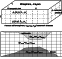Figure 1
The approach used for the calculation of the stress state in lithosphere was described for the first time by Kuang et al. . Within the framework of this approach, a lithosphere resting on an effectively liquid substrate is represented as a set of elastic layers, each characterized by constant elastic parameters and a horizontally varying density (Figure 1). The division of the lithosphere into layers and the layers into blocks corresponds to the resolution of the density model. In particular, a sufficiently fine fragmentation can be effective for the reconstruction of varying density boundaries (e.g. the Moho) in the lithosphere. The condition of equilibrium in each layer is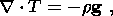(1)

where g is the gravitational acceleration, T is the stress tensor, r is density, and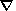is the Hamiltonian operator.

In an isotropic medium, the displacement vector u and the stress tensor are interrelated through the Hooke law: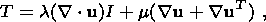(2)

where the superscript "T '' means transposition, and l and m are the Lame coefficients. Then, condition (1) can be rewritten in terms of displacements: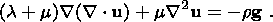(3)

The total stress field can be divided into the hydrostatic and deviatoric components. The hydrostatic component describes stresses in a horizontally homogeneous medium and is of no interest here. In order to calculate the deviatoric component, we assume that density inhomogeneities in each layer are equivalent to an additional load (with appropriate amplitude and sign) applied at the boundaries of the layer. This replacement is justified because the thickness of the layers can always be made sufficiently small, or the position of any boundary can be brought into correspondence with a concrete situation. In this case, all horizontal variations in parameters relate to boundary conditions, and the equilibrium equation without hydrostatic component is transformed into the ordinary biharmonic equation: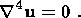(4)

We introduce a coordinate system in which the x, y and z axes are directed, respectively, eastward, northward, and upward. In this case, the basic system of boundary conditions can be written as follows: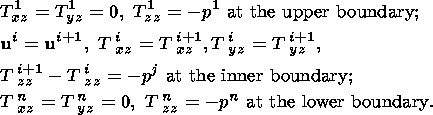(5)

Here, i is the layer number, j is the number of the boundary between the i th and ( i+1 )th layers, and pj(x,y) is the load applied at the corresponding boundary ( p1 is the topographic load, partly associated with the deformation of the plate, and pn is the anomalous pressure exerted by the mantle). The buoyancy force is incorporated as part of the load p. If concrete information on the external forces applied to the system is available, these boundary conditions can easily be modified. For example, nonzero values can be assigned to the tangential stresses at the lower boundary, if the underplating of mantle material should be taken into account.

Equation (4) with boundary conditions (5) is solved with the use of Fourier transformations by the method proposed by Kuang et al. . An infinite lithospheric plate is considered, which means that the width of the marginal areas in the region of calculations should be significantly greater than the effective thickness of the elastic plate. Using the density models of the crust and upper mantle constructed in the preceding papers of this series [Kaban, 2001, 2002], the region of calculations can always be chosen sufficiently large, a few hundred kilometers wider than the study area. Numerical experiments showed that, with a maximum effective thickness of the elastic plate of about 70 km (e.g. see [Djomani et al., 1999]), the enlargement of marginal areas by more than 250-300 km did not have any significant effect on results.

Figure 1 illustrates schematically the method used for the parametrization of a 3-D density model and converting density boundary depth variations into density variations in relatively thin plane-parallel layers. Deviations of the real density from the standard horizontally homogeneous model, described above, are calculated in each layer. The lateral variations are largest if the layer is crossed by a contrasting density boundary (e.g. the Moho). In this case, some density variations characterize the crust, and others, with an appropriate density difference, characterize the mantle. If the layer includes a boundary, an average value is calculated. Thus, density variations in upper layers account for heterogeneities in the sedimentary cover, and lower layers successively describe the solid crust and upper mantle. All density values were taken from the isostatic model of the North Eurasian lithosphere described in [Kaban, 2001]. They were complemented with density anomalies corresponding to local isostatic anomalies, i.e. the residual gravity field, which does not correspond to the isostatic density model. These additional density anomalies were calculated under the assumption that local isostatic anomalies are due to the effect of the upper 20 km of the section. Preliminary calculations showed that variations of this parameter within 10-30 km have an insignificant effect on the model stresses in the upper crust.

The following features distinguish the method, used in this work, from similar previous studies [Assameur and Mareshal, 1995; Kuang et al., 1989].

1. Previously, only the topography and Bouguer anomalies were utilized as initial parameters in calculations of stresses. This work uses the density model of the lithosphere, constructed on the basis of joint analysis of the gravity field and the data on the crust and upper mantle structure obtained by various geological and geophysical methods. This model involves density inhomogeneities at various levels that often do not correlate between each other. Trial calculations showed that stresses calculated from such a model and estimates from topography data and Bouguer anomalies differ by more than 1.5 orders of magnitude. It is also very important to incorporate the effect of real variations in depths to the Moho and isostatic gravity anomalies [Kaban and Yunga, 2000].

2. In-situ values of elastic parameters estimated from seismic velocities were used throughout the lithosphere thickness in [Assameur and Mareshal, 1995; Kuang et al., 1989]. The Lame coefficients varied from 3.8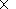10 10 Pa at the surface to 6.810 10 Pa at a depth of 100 km. In this case, the effective thickness of elastic lithosphere exceeds 120 km, which is significantly greater than the estimates derived from the study of the lithosphere response to an external load at times on the order of 10 5 years and more (e.g. [Burov and Diament, 1995]).

### 3. Modeling Results

#### Effective Rheological Characteristics of the Lithosphere

It is important to determine the effective elastic moduli best fitting the real lithosphere. Elastic models are successfully applied to analysis of media with complex rheologies, but effective values of their elastic parameters differ significantly from values measured in rocks (or determined from seismic velocities). It is assumed that, with the exception of a thin surface layer, small strains in the upper crust are approximated reasonably well by "instantaneous'' values of elastic parameters. Supposedly, the lower crust is a rheologically weakened layer with substantially smaller effective values of elastic moduli [Kusznir, 1991; Ranalli and Murphy, 1987]. In tectonically stable continental areas, the subcrustal layer can also be described in terms of a fully elastic rheology but, in tectonically active regions, a temperature of 600o C critical for olivine-bearing rocks can be exceeded even in the lower crust, drastically decreasing the effective elastic parameters [Burov and Diament, 1995].

The model used for the calculation of stresses was chosen precisely from these considerations. The main parameters of its layers are presented in Table 1. The number of the layers was chosen in a way enabling an adequate reconstruction of the inferred density model, including the variations in density boundaries. The Lame coefficients in the uppermost crustal layer to a depth of 5 km are about twice as small as the values that can be obtained by a formal conversion of seismic velocities and this fact is due to a significant fragmentation (fracturing) of the rocks. At greater depths, the Lame coefficients nearly coincide with estimates obtained by the seismic velocity conversion. In tectonically stable regions, the lower boundary of this layer reaches depths of 30-40 km but lies above a depth of 20 km in areas of high recent tectonic activity. At greater depths, effective elastic moduli gradually decrease and become close to zero at depths of about 70 km in stable regions and at about 35 km in tectonically active areas. The horizontal resolution is determined by the resolution of the initial gravity data and amounts to 10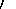15(approximately 1918 km).

It is evident that this distribution of elastic moduli is rather rough and at least considerable lateral variations in their values should exist. In order to estimate the implications of variations in these parameters, we obtained preliminary estimates from basically different models. The model stress values were found to be fairly stable in only the upper crust (5-20 km), where they vary by no more than 20% in response to variations in elastic model parameters within wide limits. However, this is valid only if large-scale blocks of the lithosphere are isostatically compensated, i.e. in the absence of sources producing long-wave components of the stress field. Since the latter condition is automatically incorporated into the density model, the calculated values should actually represent the local component of the stress field. Of course, these results are invalid in areas where the lithosphere is fractured (for example, near transform faults). In this work such areas are not considered.

#### Local Stresses in the Upper Crust of North Eurasia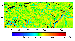Figure 2
As mentioned above, the isostatic gravity anomalies calculated in [Kaban, 2001] provide much more authentic constraints on local stresses in the upper lithosphere compared to the same anomalies estimated by other authors with the use of standard schemes of the isostatic compensation (e.g. see [Artemjev, 1975]). First, their calculation incorporated specific features of the upper crust structure (for example, thickness and density variations of low-density sediments) and, second, the isostatic compensation models fitting the real structure of lithosphere were used. Importantly, the long-wavelength component, caused by the effect of deep density inhomogeneities far below the lithosphere [Kaban, 2001], was eliminated from the total field of isostatic anomalies. The resulting local isostatic anomalies are shown in Figure 2.

As seen from Figure 2, the intensity (variability) of the field of isostatic anomalies is directly related to the degree of tectonic activity (both recent and ancient) of individual regions. The subsequent calculations account for the total effect of all variations in the density of the crust and upper mantle on the stress field.

The method and the lithosphere density model, described above, were used for the calculation of six independent components of the stress tensor at 1015grid nodes at a depth of 12.5 km. This depth was chosen on the basis of the aforementioned considerations. At greater depths, effective elastic moduli depend significantly on several factors that can vary strongly within the region studied. Preliminary tests showed that the stresses calculated in the upper crust gradually vary with depth: the short-wave component, caused by sharp lateral variations in the topography and shallow density inhomogeneities, becomes somewhat smoothed with depth. Thus, values calculated at depths of 10 to 15 km characterize the upper crustal layer as a whole and can be compared with seismicity data.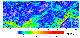Figure 3
Figure 3 shows values of the difference between the maximum and minimum principal values of the stress tensor S1-S3 (the twofold maximum tangential stress 2 t ) obtained after diagonalization of the stress tensor T. This parameter can be regarded as one of the most general characteristics of the stress state of lithosphere. As seen from Figure 3, its values reach 150 MPa, which is on the order of magnitude comparable with maximum amplitudes of stresses determined by seismic methods [Zoback, 1992]. Tectonically active areas coincide on the whole well with areas of higher tangential stresses tmax.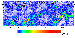Figure 4
Another important parameter characterizing the calculated field of stresses is the quantity s  2xz + s  2yz whose distribution over the study region is shown in Figure 4. Higher values of this parameter can be related to areas where subvertical movements at boundaries of lithospheric blocks are most probable and is sometimes referred to as the shearing stress. It is important to note that its value is weakly affected by the regional stress field, which enables direct comparison the calculated values with its estimates from seismic data.

Some parameters of the calculated stresses and isostatic gravity anomalies are presented in Table 2 for several tectonic structures. It is evident that the highest gradients of isostatic anomalies and the largest stresses in the upper crust concentrate in contact zones of lithospheric plates, at both continent/ocean and intracontinental boundaries. Note that these areas also include the Baikal rift zone, where the influence of regional stresses is less significant. The latter emphasizes an important role of local stresses associated with heterogeneities in the lithosphere.

### 4. Comparison of Model Stresses With Seismicity

The method, used in this work for the calculation of stresses, involves several assumptions (for example, on rheological properties of the lithosphere) that can significantly affect the results. Therefore, the comparison of model estimates with experimental data is of critical importance. Information required for such a comparison can be recovered from analysis of seismicity. It is also important to choose an area where density inhomogeneities of the lithosphere and upper mantle are a major controlling factor as compared with external forces associated with the interaction between plates.

Results of the stress calculations were compared with seismicity parameters in an area adjacent to the Baikal rift zone [Kaban and Yunga, 2000]. This area was chosen for the following reasons. First, the Baikal rift is relatively far from plate collision zones and one may suppose that the stress state in the area is controlled by density inhomogeneities of the crust and upper mantle to a much greater extent than in other areas (for example, near collision orogens). Second, the Baikal rift zone is characterized by a high seismicity level, thereby validating the comparison with results of the calculations.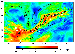Figure 5
Figure 5 shows values of the difference between the maximum and minimum principal values of the stress tensor S1-S2 (2 tmax ) in the Baikal rift region. Also shown are epicenters of M>5 earthquakes. Nearly all epicenters lie within the higher stress area and, moreover, one may state that even local features of seismically active regions correlate well with areas of higher model stresses. For example, the seismically passive northern part of Lake Baikal is characterized by relatively low stresses, whereas higher stresses are observed in its southern part.Figure 6
Figure 6 plots the relative numbers of earthquakes of M>3 and of M>5 as a function of the parameter S1-S3 (2 tmax ). As is clearly seen from these plots, the number of earthquakes becomes appreciable at stress values of about 7 MPa and remains nearly constant until values of 33-35 MPa at which the second, more abrupt increase in their number takes place. This second jump is mainly due to the rise in the number of strong earthquakes. Thus, these two stress values can be regarded as critical for earthquake generation.

Thus, even qualitative analysis of the calculated stresses with the distribution of earthquakes indicates that these stresses are actually a part of the total field of stresses in the lithosphere. The next step is quantitative comparison of the results with information that can be gained from the analysis of focal mechanisms. This task was carried out in [Kaban and Yunga, 2000]. Focal mechanisms of earthquakes in the Baikal rift zone have been investigated for more than 40 years (e.g. see [Balakina et al., 1996; Doser, 1991; Solonenko et al., 1996]. More than 3000 earthquakes have been studied; 200 of these events were characterized by individual mechanisms, and the remaining events, by group (or compound) mechanisms. These results are used in the analysis presented below.

The method consists in the comparison of predominant tendencies in the direction and type of seismotectonic deformations with patterns of stresses calculated in this work. Main features of the comparison method are as follows. First of all, the resulting tensors of stress and focal mechanisms were represented by their matrices decomposed into two main parts describing the shearing and generalized plane components. Relative intensities of these parts can be expressed in terms of a single angular parameter r: larger values of r indicate a greater role of the shearing component [Doser, 1991; Yunga, 1996].

To summarize the entire dataset, we used a formal technique based on the isometric transformation of data. In this approach, statistics of the shearing components of both focal mechanism and stress tensors are imaged as clouds of points in stereographic diagrams. Then, preferred orientations of these components are identified by eigenvalue analysis of structural matrices calculated as dyadic products of the vectors corresponding to the points of the diagrams. The results are presented in Table 3 [Kaban and Yunga, 2000].

To calculate the structural matrix corresponding to the classification diagram of stresses, the sum of 1731 dyads was used, and the number of dyads was 3271 in the case of focal mechanisms. The intensity analysis of normalized structural matrices showed that they are nonrandom on a level of at least 0.99.

Thus, we arrive at the conclusion that the calculated values of stresses in the lithosphere reliably characterize at least the local component of the complete field of stresses. We should emphasize that the values of the difference between the maximum and minimum principal values of the stress tensor S1-S3 can exceed the first critical value (7 MPa) even in stable regions (Table 2) and thereby play a significant role in the occurrence of intraplate earthquakes.

### 5. Conclusion

The stress state of the lithosphere is controlled by many factors whose significance depends on tectonic conditions of specific structures and regions. The main goal of this paper was to emphasize the importance of the study of stresses produced by density inhomogeneities in the crust and upper mantle.

The analysis carried out in this paper showed that the upper crust values of local stresses are on the order of magnitude comparable with maximum stress amplitudes determined by instrumental methods. Undoubtedly, the method used for this analysis is of limited application, particularly in areas including interplate boundaries. However, in areas remote from interaction zones of lithospheric plates, this method provides results that are consistent, at least qualitatively, with the real distribution of stresses in the lithosphere. Comparison between the distributions of earthquake sources and calculated stress amplitudes supports this conclusion.

The calculated parameters of the stress state in the lithosphere of the Baikal rift and adjacent areas agree with their estimates derived from analysis of earthquake records. Two critical values are shown to be inherent in such an important parameter of the stress field as the difference between the maximum and minimum principal values of the calculated stress field. The first critical value, amounting to 7 MPa, manifests the appearance of M>3 earthquakes and is attained even in some parts of stable platform regions. The second critical value (33-35 MPa) is associated with another abrupt rise in seismicity, largely due to strong ( M>5 ) earthquakes. Thus, one may state that, even if regional stresses play a predominant role, local stresses can serve as a triggering effect and have a significant effect on the seismicity level within limited areas where regional stresses vary weakly.

Comparison of the calculated stresses with parameters estimated from the analysis of earthquake focal mechanisms shows that the most stable characteristics of the complete stress field, obtained by this method in the upper continental crust, are associated with shearing stresses. The latter give rise to subvertical movements on local intraplate boundaries.

It is important to note that, even within stable platform regions, the model stresses can reach rather large values (up to 20 MPa, see Table 2). One may suppose that, in such areas, density inhomogeneities in the crust and upper mantle due to various factors can play a predominant role in the generation of earthquakes. This phenomenon needs additional analysis that will continue the present study.

#### Acknowledgments

Much work on comparison of the calculated stresses with focal mechanism data was done in cooperation with S. L. Yunga, to whom I particularly grateful. The method used for the stress calculation was widely discussed with Sh. A. Mukhamediev. This study was supported in part by the Russian Foundation for Basic Research, project no. 01-05-64381

### References

Artemjev, M. E. (1975), Isostasy of the USSR Territory (in Russian), 215 pp., Nauka, Moscow.

Artemjev, M. E., V. I. Bune, V. A. Dubrovsky, and N. Sh. Kambarov (1972), Seismicity and isostasy, Phys. Earth Planet. Inter., 6, 256-262.

Assameur, D. M., and J.-C. Mareshal (1995), Stress induced by topography and crustal density heterogeneities: implication for seismicity of southeastern Canada, Tectonophysics, 241, 179-192.

Balakina, L. M., A. I. Zakharova, A. G. Moskvina, and L. S. Chepkunas (1996), Natural relation of focal mechanisms of earthquakes with a geological structure of areas, Izv. Ross. Akad. Nauk, Fiz. Zemli (in Russian), (3), 33-52.

Burov, E. B., and M. Diament (1995), The effective elastic plate thickness (Te) of continental lithosphere: What does it really mean? J. Geophys. Res., 100, 3905-3927.

Djomani, Y. H. P., J. D. Fairhead, and W. L. Griffin (1999), The flexural rigidity of Fennoscandia: Reflection of the tectonothermal age of the lithospheric mantle, Earth Planet. Sci. Lett., 174, 139-154.

Doser, D. I. (1991), Faulting within the western Baikal rift as characterized by earthquake studies, Tectonophysics, 196, 87-107.

Gelfand, I. M, S. A. Guberman, V. I. Keilis-Borok, L. Knopoff, F. Press, E. Y. Ranzman, I. M. Rotwain, and A. M. Sadovsky (1983), Pattern recognition applied to earthquake epicenters in California, Workshop on Pattern Recognition and Analysis of Seismicity, CA: International Atomic Energy Agency, Vienna, Austria, International Centre for Theoretical Physics, Trieste.

Kaban, M. K. (2001), A gravitational model of the crust and upper mantle of North Eurasia, 1. Mantle and isostatic gravity anomalies, Russian J. Earth Sci., 3, (3), 143-163.

Kaban, M. K. (2002), A gravity model of the north Eurasia crust and upper mantle: 2. The Alpine-Mediterranean foldbelt and adjacent structures of the southern former USSR, Russian J. Earth Sci., 4, (1), 19-33.

Kaban, M. K., and S. L. Yunga (2000), The effect of density inhomogeneities on the stress state and seismicity of the Baikal lithosphere, Dokl. Russ. Akad. Nauk (in Russian), 371, 527-531.

Kuang, J., L. T. Long, and J. C. Mareshal (1989), Intraplate seismicity and stress in the southeastern United States, Tectonophysics, 170, 29-42.

Kusznir, N. J. (1991), The distribution of stress with depth in the lithosphere: Thermo-rheological and geodynamic constraints, Philos. Trans. R. Soc. London, 337, 95-110.

Ranalli, G., and D. C. Murphy (1987), Rheological stratification of the lithosphere, Tectonophysics, 132, 281-295.

Solonenko, A. V., N. V. Solonenko, V. I. Melnikova, and S. L. Yunga (1996), Seismicity and Seismic Regionalization of North Eurasia (in Russian), issue 2/3, pp. 363-371, Moscow.

Yunga, S. L. (1996), Seismotectonic deformations and stresses in fold belts of Neotectonic activisation of Northern Eurasia, Izv. Ross. Akad. Nauk, Fiz. Zemli (in Russian), (12), 37-58.

Zoback, M. L., et al. (1992), World stress map - maximum horizontal stress orientation, J. Geophys. Res., 97, (B8) (Application), 1992.

Load files for printing and local use. This document was generated by TeXWeb (Win32, v.1.3) on July 14, 2004.